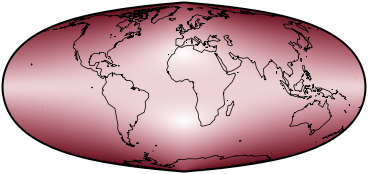## Geocart Projections

What is a projection?

Previous | Next

sinucyliParameters: Weight of sinusoidal, Weighted axis, φ₁, Vertical stretch of sinusoidal

Description

Sinucyli is a fusion of the sinusoidal and Lambert cylindrical equal-area projections.

Classifications

Pseudocylindric
Equal-area

Graticule

Meridians: Complex curves equally spaced along parallels
Parallels: Straight parallel lines
Poles: Points or lines depending on configuration
Symmetry: Bilaterally symmetric

Scale

Correct along the two parallels of latitude ±<i>φ</i>₁, which is configurable, when Vertical stretch” = 1.0.

Distortion

No distortion along the two parallels of latitude ±<i>φ</i>₁. As with any pseudocylindric, distortion increases toward outer meridians.

Parameters

Weight of sinusoidal: The fraction of weighting, from [0…1], given to sinusoidal over Lambert equal-area.
Weighted axis: Horizontal or vertical, which axis to perform the averaging between the two projections.
<i>φ</i>₁: The latitudes north and south of the equator at which the projection has no distortion.
Vertical stretch of sinusoidal: How much to stretch the sinusoidal before performing the averaging.

Limiting forms

Sinsoidal, when “Weight of sinusoidal” = 1.0, <i>φ</i>₁ = 0.0, “Weighted axis” = Horizontal, “Vertical stretch” = 1.0.
Lambert cylindrical equal-area, when “Weight of sinusoidal” = 0.0, <i>φ</i>₁ = 0.0, “Weighted axis” = Horizontal, “Vertical stretch” = 1.0.
Nell, when “Weight of sinusoidal” = 0.5, <i>φ</i>₁ = 0.0, “Weighted axis” = Horizontal, “Vertical stretch” = 1.0.

Origin

Developed by Daniel “daan” Strebe (1962–) in 2015